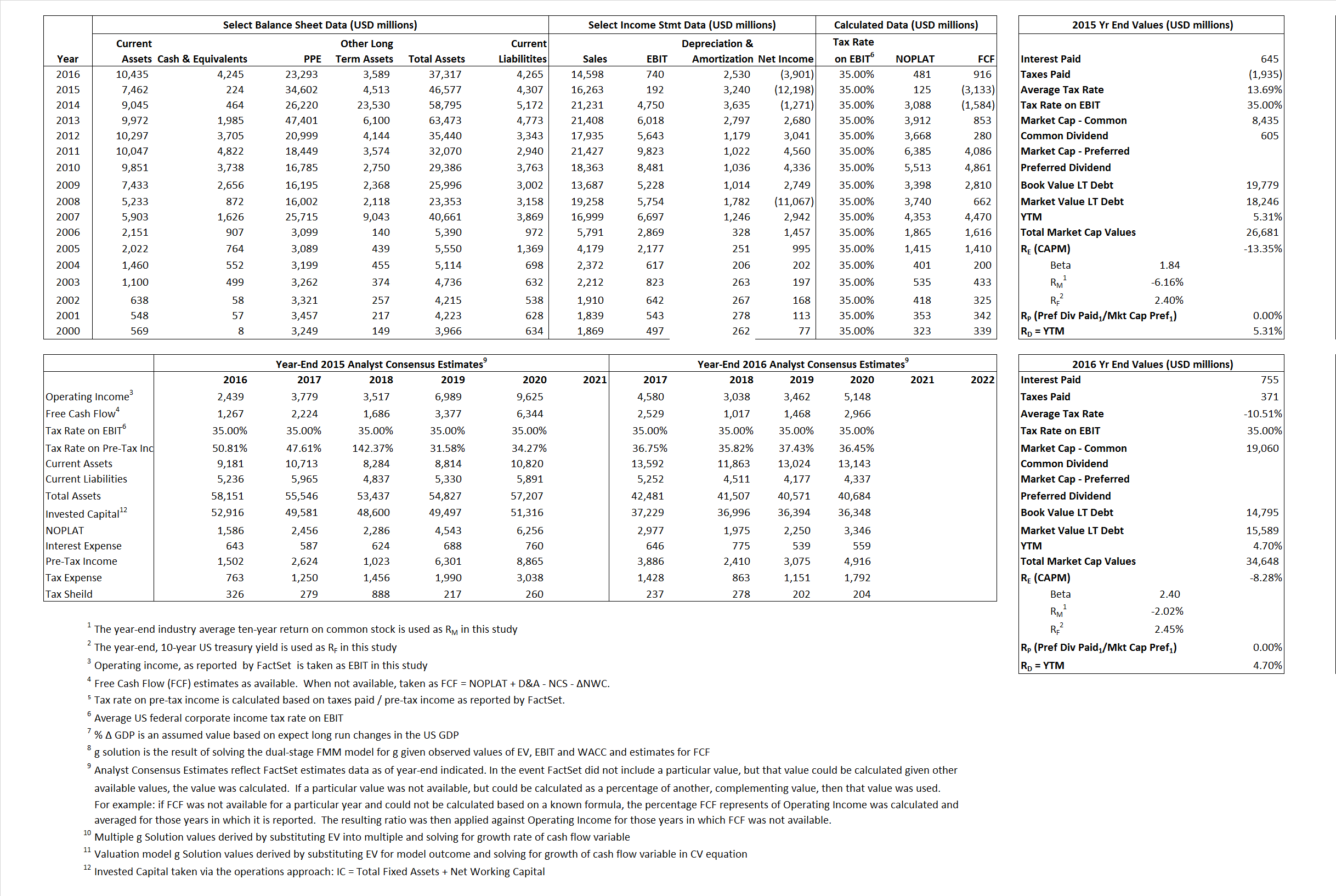# Freeport-Mcmoran

## Analyst Listing

The following analysts provide coverage for the subject firm as of May 2016:

 Broker Analyst Analyst Email Clarksons Platou Securities Jeremy Sussman jeremy.sussman@clarksons.com RBC Capital Markets Fraser Phillips fraser.phillips@rbccm.com Scotiabank GBM Orest Wowkodaw orest.wowkodaw@scotiabank.com Deutsche Bank Research Chris Terry chris.terry@db.com Stifel Nicolaus Paul Massoud pamassoud@stifel.com Jefferies Christopher LaFemina clafemina@jefferies.com BMO Capital Markets David Gagliano david.gagliano@bmo.com FBR Capital Markets & Co Lucas Pipes lpipes@fbr.com BB&T Capital Markets Garrett S. Nelson gnelson@bbandtcm.com Cowen & Company Anthony B. Rizzuto Jr. anthony.rizzuto@cowen.com Gabelli & Company Chris Mancini cmancini@gabelli.com Berenberg Fawzi Hanano fawzi.hanano.@berenberg.com Morningstar Jeffrey Stafford jeffrey.stafford@morningstar.com Argus Research William Selesky bselesky@argusresearch.com

## Primary Input Data## Derived Input Data

### Equational Form

Net Operating Profit Less Adjusted Taxes NOPLAT  125  481$NOPLAT\, =\, EBIT\, x\, (1 \,-\, Avg \,\,Tax\,\, Rate\,\, on\,\, EBIT)$
Free Cash Flow FCF (3,133) 916$FCF\,=NOPLAT\,+\,Non-Cash\,Expenses-\Delta NWC\,-\,NCS$
Tax Shield TS 88 (79)$TS\,=\,Interest\,\,Paid\,\,x\,\, Avg \,\,Tax\,\,Rate\,\, on\,\, Pre-Tax\,\, Income$
Invested Capital IC 42,270 33,052$IC\,=\,Fixed\,\,Operating\,\,Assets\,\,+\,\,Net\,\, Working\,\, Capital$
Return on Invested Capital ROIC 0.30% 1.46%$ROIC\,=\,\frac { NOPLAT }{ IC }$
Net Investment NetInv (8,113) (6,688)$NetInv\,=\,{ {IC}_{1}}-{{IC}_{0}}+Depreciation$
Investment Rate IR -6,500.80% -1,390.44%$IR\,=\,\frac {NetInv}{NOPLAT}$
Weighted Average Cost of Capital
WACCMarket  -1.09%  -2.22%$WACC\,=\,\frac { E }{ V } { R }_{ E }\,+\,\frac { P }{ V } { R }_{ P }\,+\,\frac { D }{ V } { R }_{ D }\left( 1- Avg\,\, Tax\,\,Rate\,\,on\,\,Pre-Tax\,\,Income \right)$
WACCBook       6.85%      10.53%
Enterprise value
EVMarket  26,457  30,403$EV\,=\,Market\,\,Cap\,\,Equity\,+\,\,Long\,\,Term\,\,Debt\,-\,Cash$
EVBook      27,990     29,610
Long-Run Growth
g = IR x ROIC
-19.19%    -20.23% Long-run growth rates of the income variable are used in the Continuing Value portion of the valuation models.
g = %$\Delta$ GDP      2.50%      2.50%
Margin from Operations M      1.18%     5.07%$M\,\,=\,\,\frac{EBIT}{SALES}$
Depreciation/Amortization Rate D     94.41%     77.37%$D\,\,=\,\,\frac{D+A}{EBITDA}$

## Valuation Multiple Outcomes

The outcomes presented in this study are the result of original input data, derived data, and synthesized inputs.

### model g solution

12/31/2015 12/31/2016 12/31/2015 12/31/2016 12/31/2015 12/31/2016

EV/SALES$\frac {EV}{Sales} \,= \,\frac{ROIC\, -\, g}{ROIC\,(WACC\,-\,g)}\,(1\,-\,T)\,(M)$

1.63  2.08  2.61%  43.60%  0.74% 20.87%

EV/EBITDA$\frac {EV}{EBITDA} \,= \,\frac{ROIC\, -\, g}{ROIC\,(WACC\,-\,g)}\,(1\,-\,T)\,(1\,-\,D)$

7.71 9.30 2.61% 43.60%  0.74% 20.87%

EV/NOPLAT$\frac {EV}{NOPLAT} \,= \,\frac{ROIC\, -\, g}{ROIC\,(WACC\,-\,g)}$

212.00 63.21 2.61% 43.60%  0.74% 20.87%

EV/FCFOPS$\frac {EV}{FCF_{OPS}} \,= \,\frac{ROIC\, -\, g}{ROIC\,(WACC\,-\,g)}\,(1\,-\,T)$

-8.44 33.19 2.61% 43.60%  0.74% 20.87%

EV/EBIT$\frac {EV}{EBIT} \,= \,\frac{ROIC\, -\, g}{ROIC\,(WACC\,-\,g)}\,(1\,-\,T)$

137.80 41.09 2.61% 43.60%  0.74% 20.87%

EV/IC$\frac {EV}{IC} \,= \,\frac{ROIC\, -\, g}{WACC\,-\,g}$

0.63  0.92 2.61% 43.60%  0.74% 20.87%